This file contains the source code of an exemplary application of the D-vine copula based quantile regression approach implemented in the R-package vinereg and presented in Kraus and Czado (2017): D-vine copula based quantile regression, Computational Statistics and Data Analysis, 110, 1-18. Please, feel free to address questions to daniel.kraus@tum.de.

``````library(vinereg)
library(ggplot2)
library(dplyr)
library(tidyr)
``````

# Data analysis

``````set.seed(5)
``````

We consider the data set `abalone` from the UCI Machine Learning Repository (https://archive.ics.uci.edu/ml/datasets/abalone) and focus on the female sub-population. In a first application we only consider continuous variables and fit models to predict the quantiles of weight (`whole`) given the predictors `length`, `diameter`, and `height`.

``````data(abalone, package = "PivotalR")
abalone_f <- abalone %>%
dplyr::filter(sex == "F") %>%        # select female abalones
dplyr::select(-id, -sex) %>%         # remove id and sex variables
dplyr::filter(height < max(height))  # remove height outlier
``````
``````pairs(abalone_f, pch = ".")
``````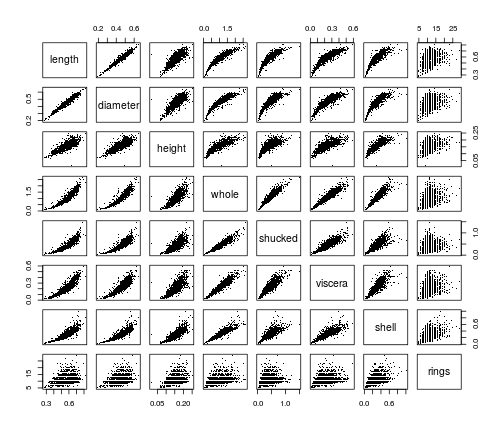# D-vine regression models

## Parametric D-vine quantile regression

We consider the female subset and fit a parametric regression D-vine for the response weight given the covariates len, diameter and height (ignoring the discreteness of some of the variables). The D-vine based model is selected sequentially by maximizing the conditional log-likelihood of the response given the covariates. Covariates are only added if they increase the (possibly AIC- or BIC-corrected) conditional log-likelihood.

We use the function `vinereg()` to fit a regression D-vine for predicting the response weight given the covariates `length`, `diameter`, and `height`. The argument `family_set` determines how the pair-copulas are estimated. We will only use one-parameter families and the t copula here. The `selcrit` argument specifies the penalty type for the conditional log-likelihood criterion for variable selection.

``````fit_vine_par <- vinereg(
whole ~ length + diameter + height,
data = abalone_f,
family_set = c("onepar", "t"),
selcrit = "aic"
)
``````

The result has a field `order` that shows the selected covariates and their ranking order in the D-vine.

``````fit_vine_par\$order
``````
``````##  "length"   "height"   "diameter"
``````

The field `vine` contains the fitted D-vine, where the first variable corresponds to the response. The object is of class `"vinecop_dist"` so we can use `rvineocpulib`'s functionality to summarize the model

``````summary(fit_vine_par\$vine)
``````
``````## # A data.frame: 6 x 11
##  tree edge conditioned conditioning var_types family rotation  parameters
##     1    1        1, 2                    c,c gumbel      180         5.2
##     1    2        2, 4                    c,c gumbel      180         2.4
##     1    3        4, 3                    c,c gumbel      180         2.4
##     2    1        1, 4            2       c,c      t        0 0.45, 21.87
##     2    2        2, 3            4       c,c      t        0  0.91, 4.68
##     3    1        1, 3         4, 2       c,c      t        0  0.31, 7.40
##  df  tau loglik
##   1 0.81   1629
##   1 0.58    672
##   1 0.59    709
##   2 0.30    147
##   2 0.73   1193
##   2 0.20     72
``````

We can also plot the contours of the fitted pair-copulas.

``````contour(fit_vine_par\$vine)
``````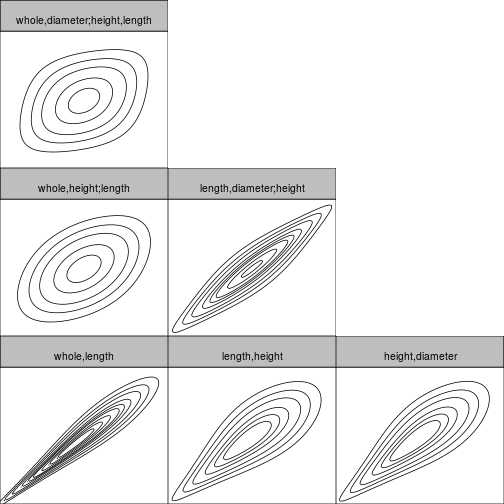## Estimation of corresponding conditional quantiles

In order to visualize the predicted influence of the covariates, we plot the estimated quantiles arising from the D-vine model at levels 0.1, 0.5 and 0.9 against each of the covariates.

``````# quantile levels
alpha_vec <- c(0.1, 0.5, 0.9)
``````

We call the `fitted()` function on `fit_vine_par` to extract the fitted values for multiple quantile levels. This is equivalent to predicting the quantile at the training data. The latter function is more useful for out-of-sample predictions.

``````pred_vine_par <- fitted(fit_vine_par, alpha = alpha_vec)
# equivalent to:
# predict(fit_vine_par, newdata = abalone.f, alpha = alpha_vec)
``````
``````##         0.1       0.5       0.9
## 1 0.6717860 0.7667223 0.8716322
## 2 0.6968823 0.7903849 0.9014626
## 3 0.6794480 0.7843661 0.8914415
## 4 0.7754026 0.8800504 0.9976871
## 5 0.5950430 0.6996904 0.8273507
## 6 0.6755811 0.7729168 0.8860188
``````

To examine the effect of the individual variables, we will plot the predicted quantiles against each of the variables. To visualize the relationship more clearly, we add a smoothed line for each quantile level. This gives an estimate of the expected effect of a variable (taking expectation with respect to all other variables).

``````plot_effects(fit_vine_par)
``````
``````## `geom_smooth()` using method = 'gam' and formula 'y ~ s(x, bs = "cs")'
``````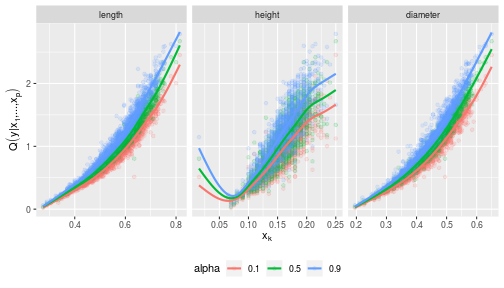The fitted quantile curves suggest a non-linear effect of all three variables.

## Comparison to the benchmark model: linear quantile regression

This can be compared to linear quantile regression:

``````pred_lqr <- pred_vine_par
for (a in seq_along(alpha_vec)) {
my.rq <- quantreg::rq(
whole ~ length + diameter + height,
tau = alpha_vec[a],
data = abalone_f
)
pred_lqr[, a] <- quantreg::predict.rq(my.rq)
}

plot_marginal_effects <- function(covs, preds) {
cbind(covs, preds) %>%
tidyr::gather(alpha, prediction, -seq_len(NCOL(covs))) %>%
dplyr::mutate(prediction = as.numeric(prediction)) %>%
tidyr::gather(variable, value, -(alpha:prediction)) %>%
ggplot(aes(value, prediction, color = alpha)) +
geom_point(alpha = 0.15) +
geom_smooth(method = "gam", formula = y ~ s(x, bs = "cs"), se = FALSE) +
facet_wrap(~ variable, scale = "free_x") +
ylab(quote(q(y* "|" * x * ",...," * x[p]))) +
xlab(quote(x[k])) +
theme(legend.position = "bottom")
}
plot_marginal_effects(abalone_f[, 1:3], pred_lqr)
``````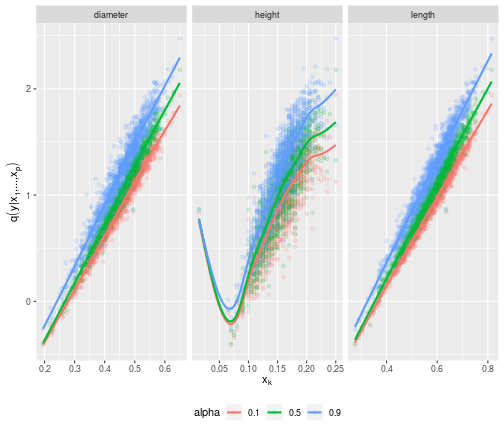## Nonparametric D-vine quantile regression

We also want to check whether these results change, when we estimate the pair-copulas nonparametrically.

``````fit_vine_np <- vinereg(
whole ~ length + diameter + height,
data = abalone_f,
family_set = "nonpar",
selcrit = "aic"
)
fit_vine_np
``````
``````## D-vine regression model: whole | length, height, diameter
## nobs = 1306, edf = 95.83, cll = 1207.07, caic = -2222.48, cbic = -1726.57
``````
``````contour(fit_vine_np\$vine)
``````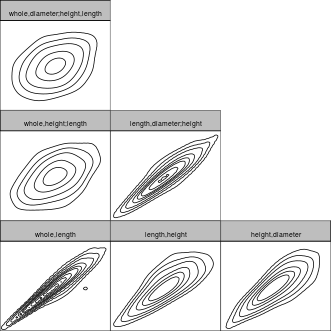Now only the length and height variables are selected as predictors. Let's have a look at the marginal effects.

``````plot_effects(fit_vine_np, var = c("diameter", "height", "length"))
``````
``````## `geom_smooth()` using method = 'gam' and formula 'y ~ s(x, bs = "cs")'
``````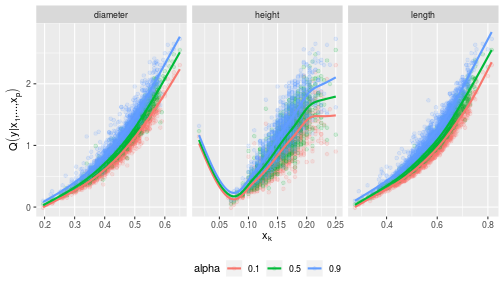The effects look similar to the parametric one, but slightly more wiggly. Note that even the diameter was not selected as a covariate, it's marginal effect is captured by the model. It just does not provide additional information when height and length are already accounted for.

## Discrete D-vine quantile regression

To deal with discrete variables, we use the methodology of Schallhorn et al. (2017). For estmiating nonparametric pair-copulas with discrete variable(s), jittering is used (Nagler, 2017).

We let `vinereg()` know that a variable is discrete by declaring it `ordered`.

``````abalone_f\$rings <- as.ordered(abalone_f\$rings)
fit_disc <- vinereg(rings ~ ., data = abalone_f, selcrit = "aic")
fit_disc
``````
``````## D-vine regression model: rings | shell, shucked, whole, viscera, height, diameter, length
## nobs = 1306, edf = 12, cll = -2763.64, caic = 5551.29, cbic = 5613.39
``````
``````plot_effects(fit_disc)
``````
``````## `geom_smooth()` using method = 'loess' and formula 'y ~ x'
``````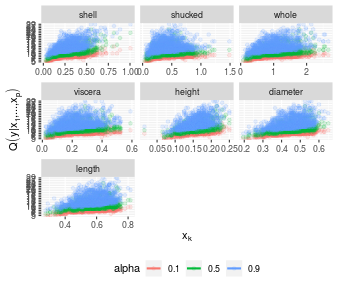# References

Kraus and Czado (2017), D-vine copula based quantile regression, Computational Statistics and Data Analysis, 110, 1-18

Nagler (2017), A generic approach to nonparametric function estimation with mixed data, Statistics & Probability Letters, 137:326–330

Schallhorn, Kraus, Nagler and Czado (2017), D-vine quantile regression with discrete variables, arXiv preprint